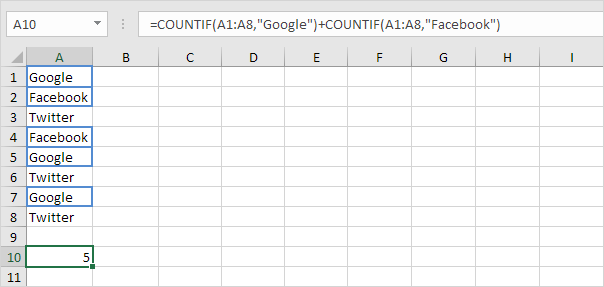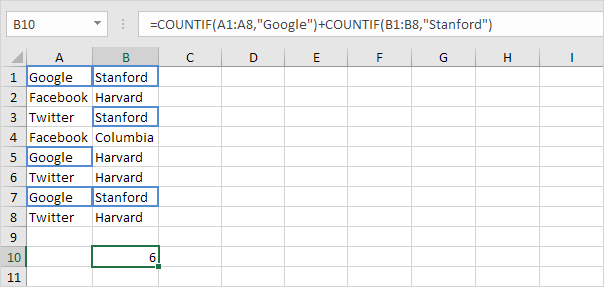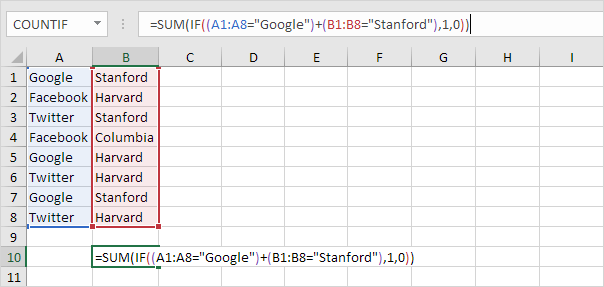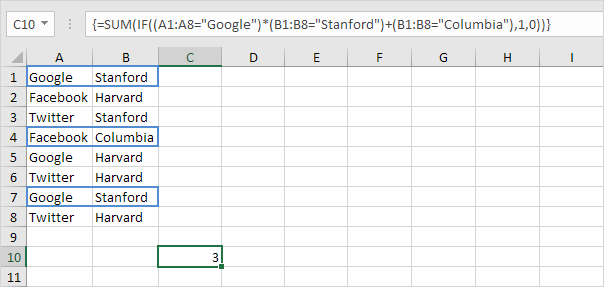# Count with Or Criteria

Counting with Or criteria in Excel can be tricky. This article shows several easy to follow examples.

1. We start simple. For example, we want to count the number of cells that contain Google or Facebook (one column).2a. However, if we want to count the number of rows that contain Google or Stanford (two columns), we cannot simply use the COUNTIF function twice (see the picture below). Rows that contain Google and Stanford are counted twice, but they should only be counted once. 4 is the answer we are looking for.2b. What we need is an array formula. We use the IF function to check if Google or Stanford occurs.Explanation: TRUE = 1, FALSE = 0. For row 1, the IF function evaluates to IF(TRUE+TRUE,1,0), IF(2,1,0), 1. So the first row will be counted. For row 2, the IF function evaluates to IF(FALSE+FALSE,1,0), IF(0,1,0), 0. So the second row will not be counted. For row 3, the IF function evaluates to IF(FALSE+TRUE,1,0), IF(1,1,0), 1. So the third row will be counted, etc.

2c. All we need is a SUM function that counts these 1's. To achieve this (don't be overwhelmed), we add the SUM function and replace A1 with A1:A8 and B1 with B1:B8.2d. Finish by pressing CTRL + SHIFT + ENTER.Note: the formula bar indicates that this is an array formula by enclosing it in curly braces {}. Do not type these yourself. They will disappear when you edit the formula. In Excel 365 or Excel 2021, finish by simply pressing Enter. You won't see curly braces.

Explanation: the range (array constant) created by the IF function is stored in Excel's memory, not in a range. The array constant looks as follows:

{1;0;1;0;1;0;1;0}

This array constant is used as an argument for the SUM function, giving a result of 4.

3. We can go one step further. For example, we want to count the number of rows that contain (Google and Stanford) or Columbia.Go to Next Chapter: Sort